# Two generalizations of Liouville λ function

André Pierro de Camargo
Notes on Number Theory and Discrete Mathematics
Print ISSN 1310–5132, Online ISSN 2367–8275
Volume 29, 2023, Number 1, Pages 30–39
DOI: 10.7546/nntdm.2023.29.1.30-39

## Details

### Authors and affiliations

André Pierro de Camargo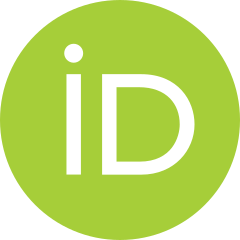Federal University of the ABC region, Brazil

### Abstract

We study the properties of two classes of functions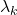and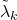that generalize the Liouville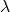function, including some equivalencies between the Riemann hypothesis and some assertions about the asymptotic behavior of the summatory functions ofand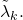Similar results are obtained for the generalization of the Möbius function considered by Tanaka.

### Keywords

• Liouville function
• Möbius function
• Prime Number Theorem
• Riemann Hypothesis

• 11N56
• 11N99

### References

1. Apostol, T. M. (1976). Introduction to Analytic Number Theory. New York: Springer–Verlag.
2. Apostol, T. M. (1970). Möbius functions of order 𝑘. Pacific Journal of Mathematics, 32(1), 21–27.
3. Camargo, A. (2021). Dirichlet matrices: Determinants, permanents and the Factorisatio Numerorum problem. Linear Algebra and Its Applications, 628, 115–129.
4. Fujisawa, Y. H. (2014). On Mobius and Liouville functions of order k. arXiv:1305.6015v2 [math.NT].
5. Humphries, P. (2013). The distribution of weighted sums of the Liouville function and Pólya’s conjecture. Journal of Number Theory, 133, 545–582.
6. Moser, L., & MacLeod. R. A. (1966). The error term for square-free integers. Canadian Mathematical Bulletin, 9(3), 303–306.
7. Panaitopol, L. (2001). Some properties of Liouville’s function. Bulletin Mathematique de la Societe des Sciences Mathematiques de Roumanie, 92(4), 365–370.
8. Pappalardi, F. (2005). A survey on 𝑘-freeness. In: S. D. Adhikari, R. Balasubramanian, & K. Srinivas (Eds.), Number Theory. Lecture Notes Series, Vol. 1, pp. 71–88. Mysore: Ramanujan Mathematical Society.
9. Tanaka, M. (1980). On Möbius and allied functions. Tokyo Journal of Mathematics, 3(2), 215–218.

### Manuscript history

• Revised: 15 December 2022
• Accepted: 10 February 2023
• Online First: 13 February 2023Ⓒ 2023 by the Author.